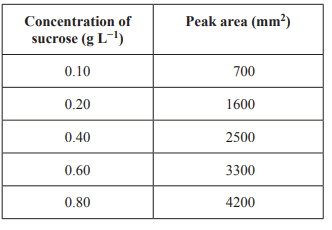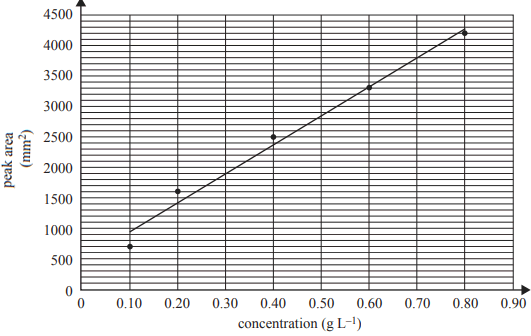.
 Chromatography (2019 VCE) 1) A compound has the molecular formula C4H9Cl. Which type of chemical analysis would be most useful in determining whether this compound has a stereoisomer? A. mass spectrometry B. infra-red spectroscopy C. high-performance liquid chromatography D. nuclear magnetic resonance spectroscopy Solution2 )High-performance liquid chromatography (HPLC) was used to determine the sucrose concentration in a sample taken from a can of soft drink. Standard solutions were made up using pure sucrose and deionised water. A 1 mL sample of each standard solution was injected into the HPLC column and its peak area was recorded, as shown in the table below.The experimental results (shown as dots) and a calibration line of the concentration of sucrose against peak area are shown in the graph below.A 5.0 mL sample of the soft drink was diluted to 100 mL in a volumetric flask. A 10.0 mL aliquot of this solution was transferred to a 250 mL volumetric flask and filled up to the calibration mark using deionised water. A sample of this solution was injected into the HPLC column. The peak area of the sample solution at the same retention time and under the same conditions as those used to determine the calibration line was found to be 1900 mm2. i. Determine the sucrose content of the sample tested in the HPLC, in grams per litre. Solution   ii. Calculate the percentage mass/volume (% m/v) of sucrose in the 5.0 mL sample of soft drink.iii. The can used to obtain the sample contained 330 mL of soft drink. Assuming that the only sugar in the soft drink is sucrose, calculate the mass of sucrose in the can of soft drink. Solutionb) Based on the results obtained, is the experimental method valid? Give your reasoning. Solution c) Sucrose and aspartame are types of sweeteners. Use your understanding of chemistry to explain why some people replace sugar with aspartame. In your answer, compare sucrose and aspartame in terms of: • metabolic reactions • glycaemic indices • energy content. Solution